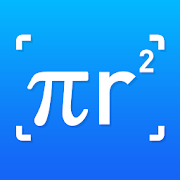Photo Mathematics - Math Solver , Photo CalculatorEveryone
841
Photo Mathematics - Math Solver , Photo Math - App to help you scan photos, solve math problems, learn math.

Photo Mathematics - Math Solver, photomath is a tool that helps learning math easier than ever through scanning threads and Photo Calculator - Math Solver, Math Calculator will return results and step-by-step solutions most accurately for you.

Math Solver, Math Scanner Answer - Application that helps you learn math and solve difficult problems , your phone can become Math scanner to solve math problem and immediately receive the photo calculator answers , Photo math.

We develop a creative way to solve maths problems. Are you still annoyed at doing maths homework by hand or knocking the calculator one by one? Now with Snap math function provided by Photo Calculator - Math Solver, Math Calculator , you just need to take a picture by camera for the complex maths homework and you can get the solutions immediately. photomath - Photo Calculator just takes you one step to solve an equations or a maths problem.

დ Features of SMART CALCULATOR & PHOTO MATHEMATICS დ
Scientific Calculator - Camera calculator simple to grasp and easy to use the usual arithmetic functions and exponentiation.Math Graph in polar coordinates.
✓ The Photo Calculator app calculates math problems with cameras quickly and accurately.
Math Problem Solver can solve such problems as: exponential equation, system of equations, integration, mathematical graphs, computations with scientific constants ...
photomath - Math Calculator can draw mathematical graphs based on scanned equations.
✓ Save your calculation history, making it easy to find difficult math problems.
✓ If your phone's camera is not working. Don't worry, because the Math Calculator can be entered manually, with lots of functions and constants supported
Photo Mathematics also contains manual math calculator, Math Graph parametric solve equations.

Complete the difficult math problems with Photo Calculator - Math Solver ,photomath.

დ Function of MATH PHOTO CALCULATOR დ
Photo Mathematics - Math Calculator: Based on casio 570 with math solution
Equation calculator : Solve your math homework efficiently.
Scan the Calculated Photo - Math Scanner Answer : Scan math problems and analyze the best solutions for problems.
Photo Calculator - Math Photo : Good math homework helper which solves math problems by taking photo.

photomath & Photo calculator - Math Scanner Answer:
Scan the problem with the camera ( Math Scan ). After scanning, Photo Calculator( Math Photo) will analyze the solution for the fastest and most accurate. Next is to display each step and save the way to easily find it again.

Equation calculator - Math Photo & Math Scanner Answer
With smart calculator, you just need to enter the formula and the equation calc will give you answers automatically.There’s a professional equation calculator specially designed for students who need to do difficult equations. What’s more, you can do decimal calculation and power calculation in the equation calculator.

We provide a mathematics solutions in a creative way. While you are doing maths home work, try our calculator so that you can solve maths problems more easily by taking photo. Both basic maths problem and equations are suitable.

Photo Mathematics - Math Solver, Math Calculator is an easy to use application for all students. Use our Math app to snap a pic and instantly get the solution with the steps! And, our Photo Calculator - Math Solver, Math Calculator is FREE!

Thank you very much for using our app!
Collapse

Reviews

Review Policy
4.7
841 total
5
4
3
2
1

Updated
June 27, 2019
Size
12M
Installs
50,000+
Current Version
1.1
Requires Android
4.1 and up
Content Rating
Everyone
Permissions
Offered By
Music Awesome App